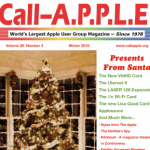# Number Bases

By Lynne Ryder
Australian Apple Review Vol 4 No 3 PP32-33

Another topic which often causes difficulty for students, yet lends itself elegantly to the computer, is number bases. I am

including two short programs – the first written by one of my students, Sandi Steeple which generates numbers for bases less than 10, while the second comes from an old, but still very useful book, ‘Fifty Basic Exercises’ by Jean-Pierre Lamoiter (published by Sybex) and generates numbers for bases greater than 10. Both demonstrate how an understanding of computers and Basic can add clarity to some otherwise tedious topics.

Number systems are built upon some base number. For us this happens, by chance or by the fact that we are pentadactyl and have two hands, to be the number 10.

Counting in base ten requires little thought for most of us. We know that there are units, tens, hundreds and so on, but we don’t need to make the mental effort to think that the integer following 99will sequential- ly have a one, a zero and a zero – that it will no longer be in the tens and units, but now will spill over into hundreds.

This should have been instilled back in kindergarten but what we may not have learnt at the time is that units represent numbers multiplied by 10 to the power of zero, tens represent numbers multiplied by 10 to the power of 1, hundreds represent numbers multiplied by 10 to the power of 2, and so on again. For example, the number six thousand seven hundred and eighty nine is (and I’ll use A to signify ‘to the power of’ and * to signify ‘multiplied by’) 6*10^3plus 7*10^2 plus 8*10^1 plus 9*10^0.

When we look at other bases other than ten, our minds are less flexible. This is understandable because we need to make some translations. The common question such as ‘What is 51 base 5?’,requires thought in base 5 while working with base 10 conventions. A more fruitful method is to first introduce counters of some description, physically set up ‘columns’ and then share the counters into the columns. Following some hands on, the same logic can be applied as for the base 10 numbers; 51 in base 5 equals, 2*5^2 plus0*5^1 plus 1*5^0, or put another way, it equals (201) base 5.

But why look at bases in the first place? Obviously a knowledge of bases is not essential to count in our number system. But, unlike subjects such as ancient history, different base number systems reused all around us in everyday life. The nearest example is the Apple in front of me. The machine works in binary (base 2) for all its processing, while if I were to go into the monitor and enter machine code, it would be in hex or base 16. If you have access to an Apple now and have the ] prompt, type CALL -151 and hit RETURN several times. What you see is hex.

The two programs following considerably simplify the thought processes when determining what decimal numbers will be in a number of different bases. This is a very interesting area, so let your students have a try at programming before showing them these two of the many alternatives possible.

``````10 HOME : VTAB 4
20 PRINT "This program will convert a range of"
30 PRINT "numbers to base N, where 2 <=N< 10"
40 DIM A(15)
50 B\$ = "0123456789ABCDEFGHIJKLMN"
60 PRINT "The new base";
70 INPUT B
80 PRINT "First and last number to convert"
90 INPUT F, L
100 FOR I = F TO L
110 PRINT
120 GOSUB 210
130 REM PRINT A TABLE ENTRY
140 PRINT I; TAB (6);
150 FOR D = J TO 1 STEP -1
160 PRINT MID\$ (B\$, A (D) +
170 NEXT D
180 NEXT I
190 STOP
200 REM BASE CONVERSION SUBROUTINE
210 I1 = I
220 J = 1
230 Q = INT (I1/B)
240 R = I1 - Q * B
250 I1 = Q
260 A (J) = R
270 J = J + 1
280 IF Q > = B THEN 230
290 A (J) = Q
300 RETURN
310 END ``````

#### About the Author#### A.P.P.L.E.

The A.P.P.L.E. Website is run by the Apple Pugetsound Program Library Exchange Users Group and is open to all Apple and Macintosh fans and their friends.